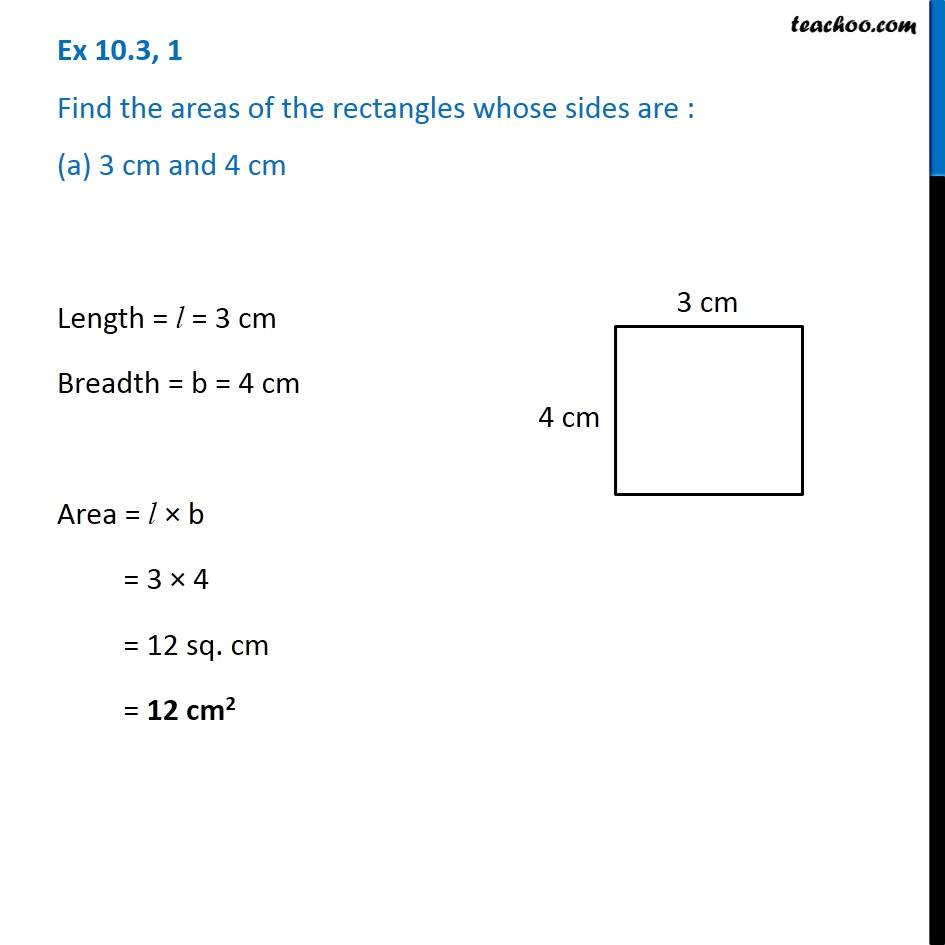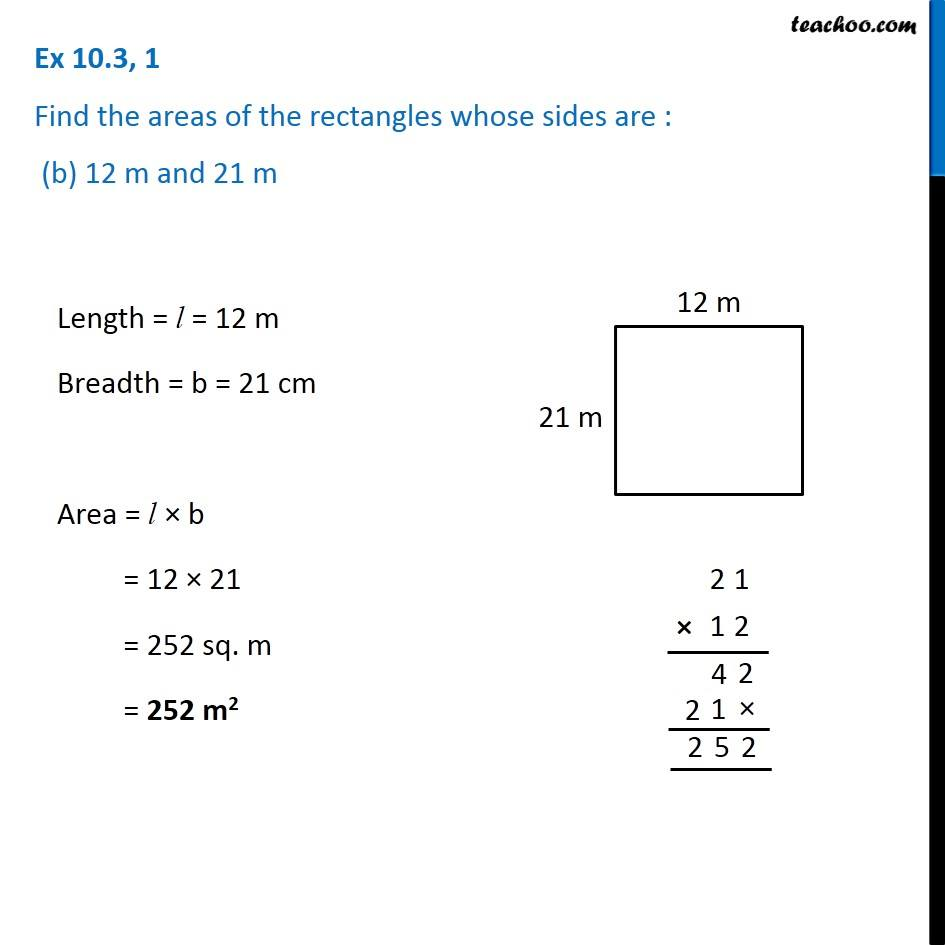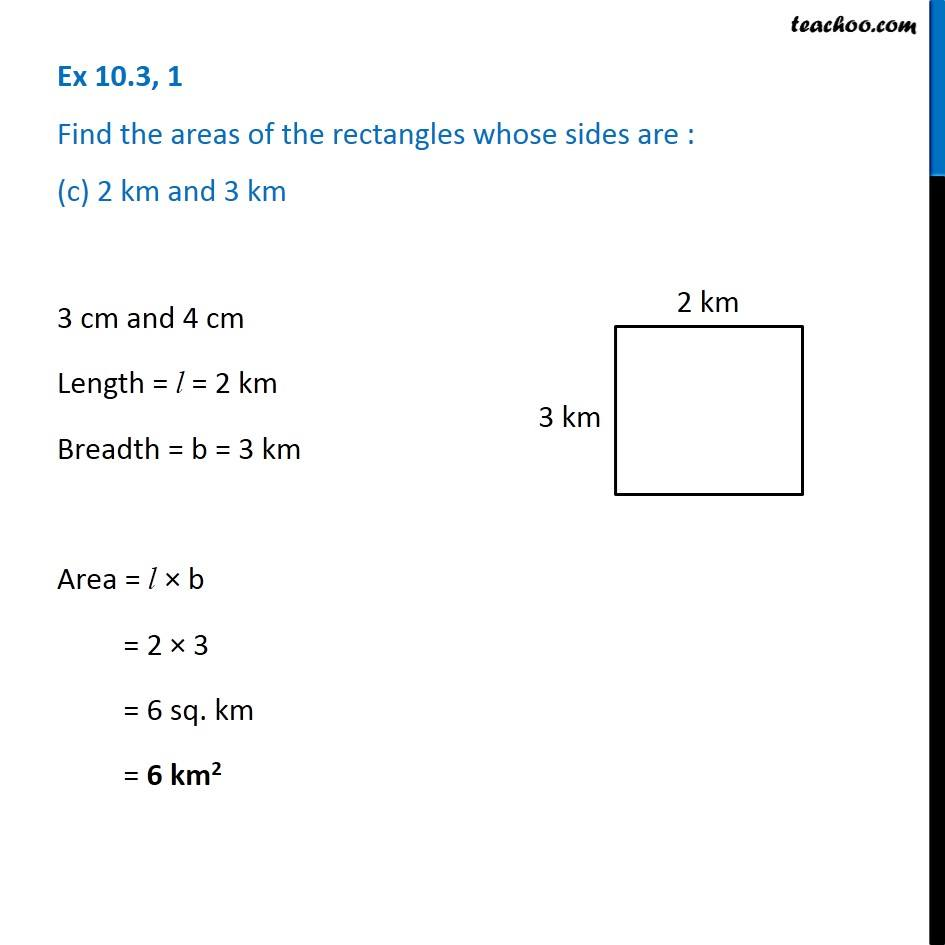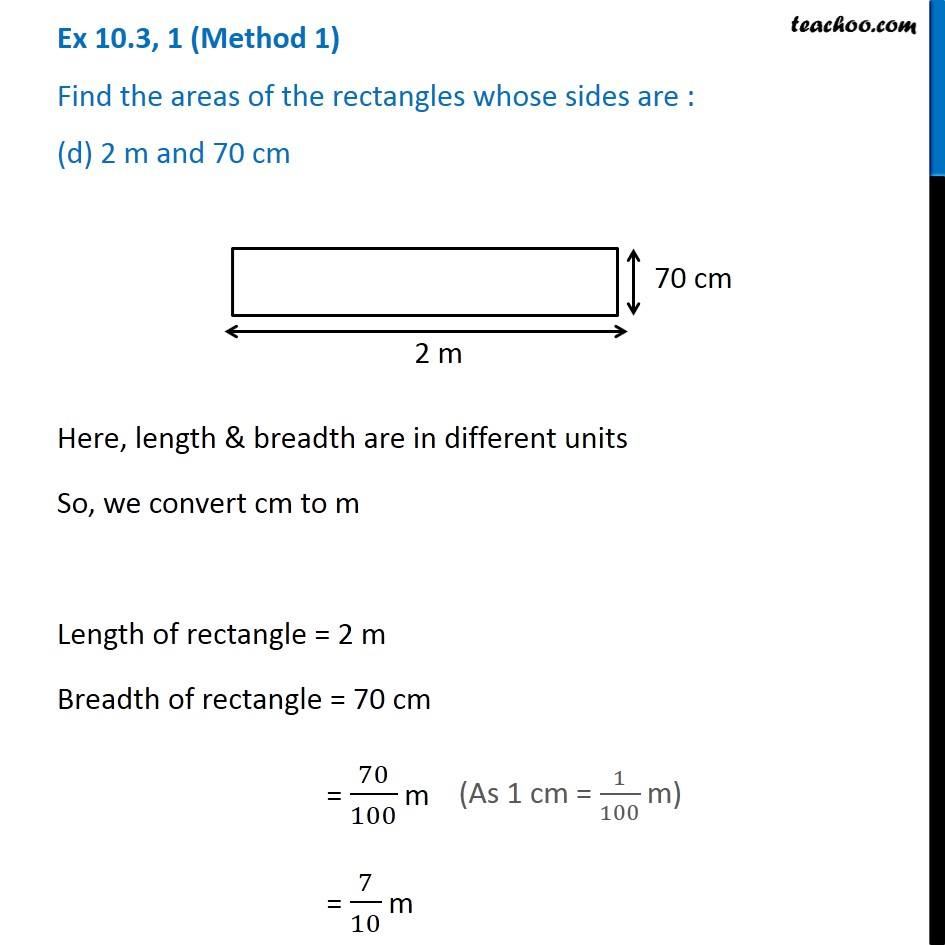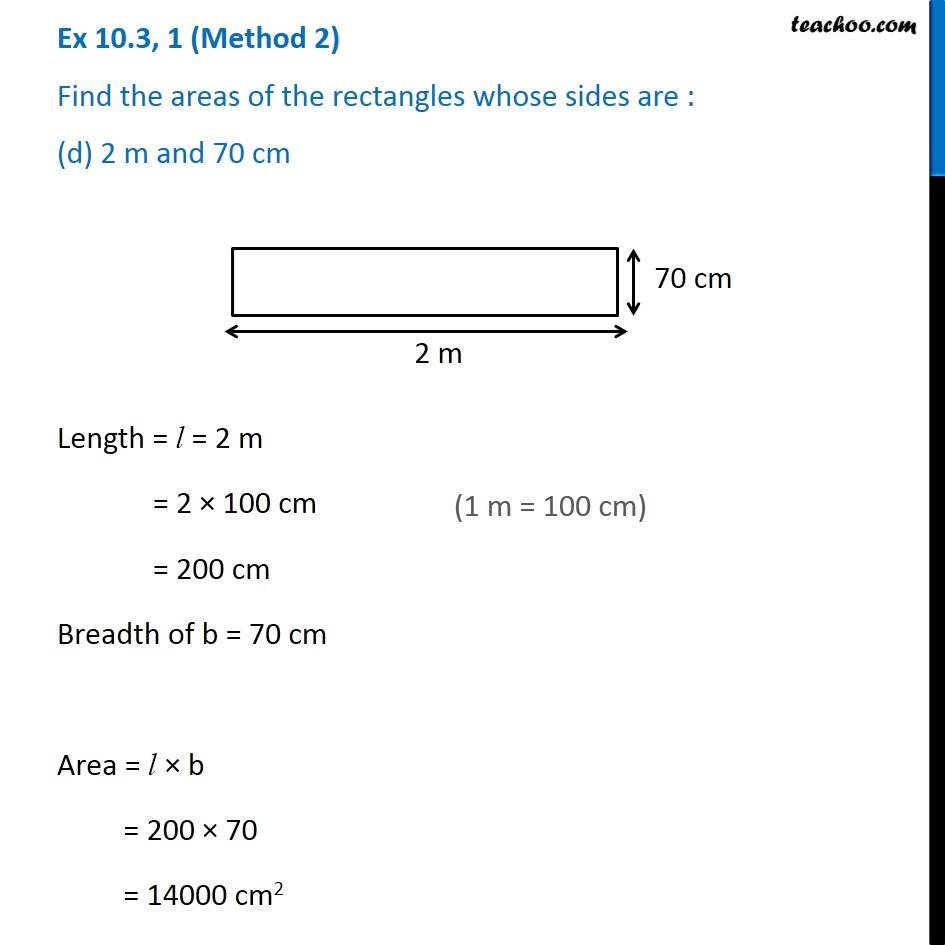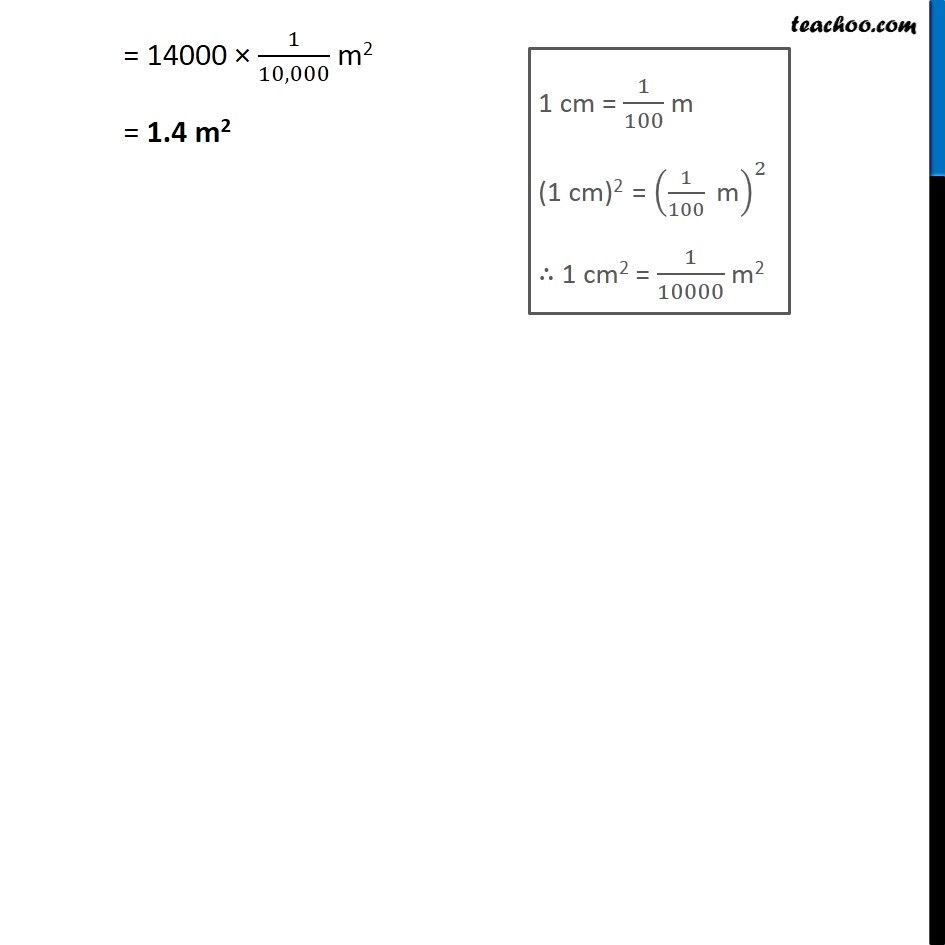Subscribe to our Youtube Channel - https://you.tube/teachoo

1. Chapter 10 Class 6 Mensuration
2. Serial order wise
3. Ex 10.3

Transcript

Ex 10.3, 1 Find the areas of the rectangles whose sides are : (a) 3 cm and 4 cm Length = l = 3 cm Breadth = b = 4 cm Area = l × b = 3 × 4 = 12 sq. cm = 12 cm2 Ex 10.3, 1 Find the areas of the rectangles whose sides are : (b) 12 m and 21 m Length = l = 12 m Breadth = b = 21 cm Area = l × b = 12 × 21 = 252 sq. m = 252 m2 Ex 10.3, 1 Find the areas of the rectangles whose sides are : (c) 2 km and 3 km 3 cm and 4 cm Length = l = 2 km Breadth = b = 3 km Area = l × b = 2 × 3 = 6 sq. km = 6 km2 Ex 10.3, 1 (Method 1) Find the areas of the rectangles whose sides are : (d) 2 m and 70 cm Here, length & breadth are in different units So, we convert cm to m Length of rectangle = 2 m Breadth of rectangle = 70 cm = 70/100 m = 7/10 m (As 1 cm = 1/100 m) Area of rectangle = Length × Breadth = 2 × 7/10 = 14/10 = 1.4 sq. m = 1.4 m2 Ex 10.3, 1 (Method 2) Find the areas of the rectangles whose sides are : (d) 2 m and 70 cm Length = l = 2 m = 2 × 100 cm = 200 cm Breadth of b = 70 cm Area = l × b = 200 × 70 = 14000 cm2 (1 m = 100 cm) = 14000 × 1/10,000 m2 = 1.4 m2 1 cm = 1/100 m (1 cm)2 = (1/100 " m" )^2 ∴ 1 cm2 = 1/10000 m2

Ex 10.3

Chapter 10 Class 6 Mensuration
Serial order wise